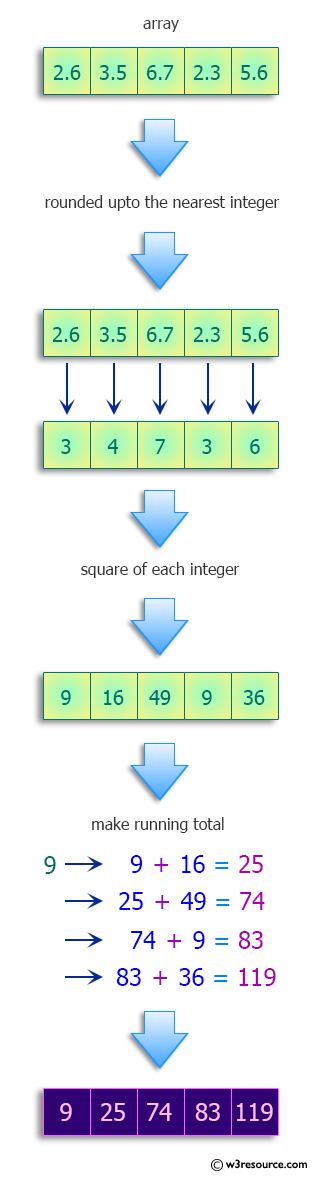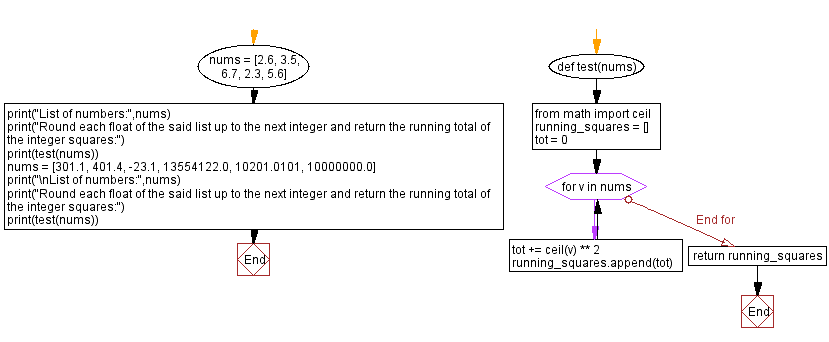﻿ Python: Round each float in a list of numbers up to the next integer and return the running total of the integer squares - w3resource# Python: Round each float in a list of numbers up to the next integer and return the running total of the integer squares

## Python Programming Puzzles: Exercise-80 with Solution

Write a Python program to round each float in a given list of number up to the next integer and return the running total of the integer squares.

```Input:
[2.6, 3.5, 6.7, 2.3, 5.6]
Output:
[9, 25, 74, 83, 119]

Input:
[301.1, 401.4, -23.1, 13554122.0, 10201.0101, 10000000.0]
Output:
[91204, 252808, 253337, 183714223444221, 183714327525025, 283714327525025]
```

Pictorial Presentation:Sample Solution:

Python Code:

``````#License: https://bit.ly/3oLErEI

def test(nums):
from math import ceil
running_squares = []
tot = 0
for v in nums:
tot += ceil(v) ** 2
running_squares.append(tot)
return running_squares

nums = [2.6, 3.5, 6.7, 2.3, 5.6]
print("List of numbers:",nums)
print("Round each float of the said list up to the next integer and return the running total of the integer squares:")
print(test(nums))
nums = [301.1, 401.4, -23.1, 13554122.0, 10201.0101, 10000000.0]
print("\nList of numbers:",nums)
print("Round each float of the said list up to the next integer and return the running total of the integer squares:")
print(test(nums))
``````

Sample Output:

```List of numbers: [2.6, 3.5, 6.7, 2.3, 5.6]
Round each float of the said list up to the next integer and return the running total of the integer squares:
[9, 25, 74, 83, 119]

List of numbers: [301.1, 401.4, -23.1, 13554122.0, 10201.0101, 10000000.0]
Round each float of the said list up to the next integer and return the running total of the integer squares:
[91204, 252808, 253337, 183714223444221, 183714327525025, 283714327525025]
```

Flowchart:## Visualize Python code execution:

The following tool visualize what the computer is doing step-by-step as it executes the said program:

Python Code Editor :

Have another way to solve this solution? Contribute your code (and comments) through Disqus.

What is the difficulty level of this exercise?

Test your Programming skills with w3resource's quiz.

﻿

## Python: Tips of the Day

Clamps num within the inclusive range specified by the boundary values x and y:

Example:

```def tips_clamp_num(num,x,y):
return max(min(num, max(x, y)), min(x, y))
print(tips_clamp_num(2, 4, 6))
print(tips_clamp_num(1, -1, -6))
```

Output:

```4
-1
```